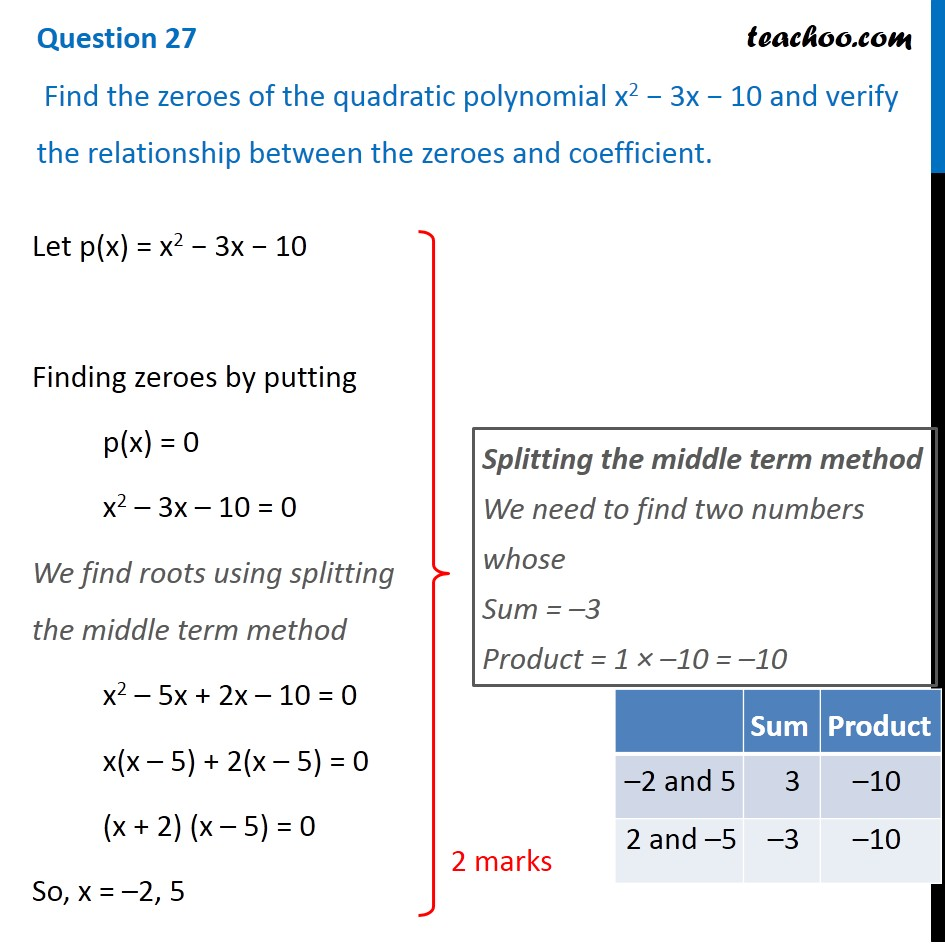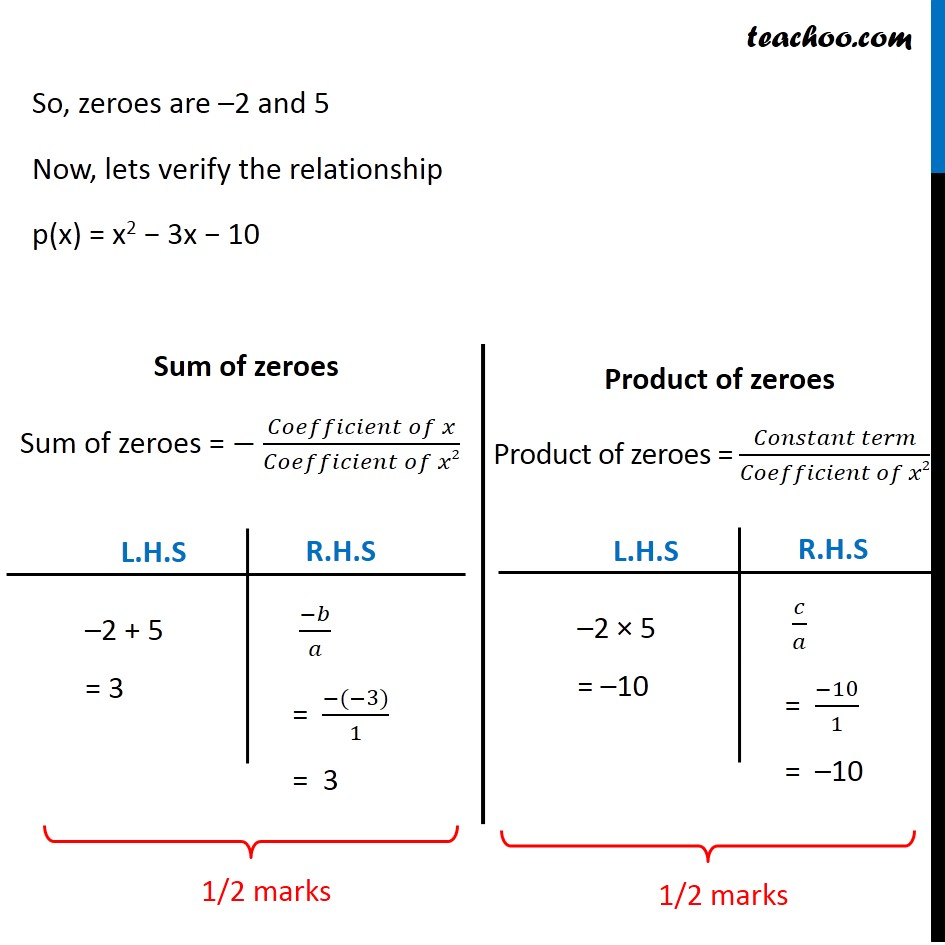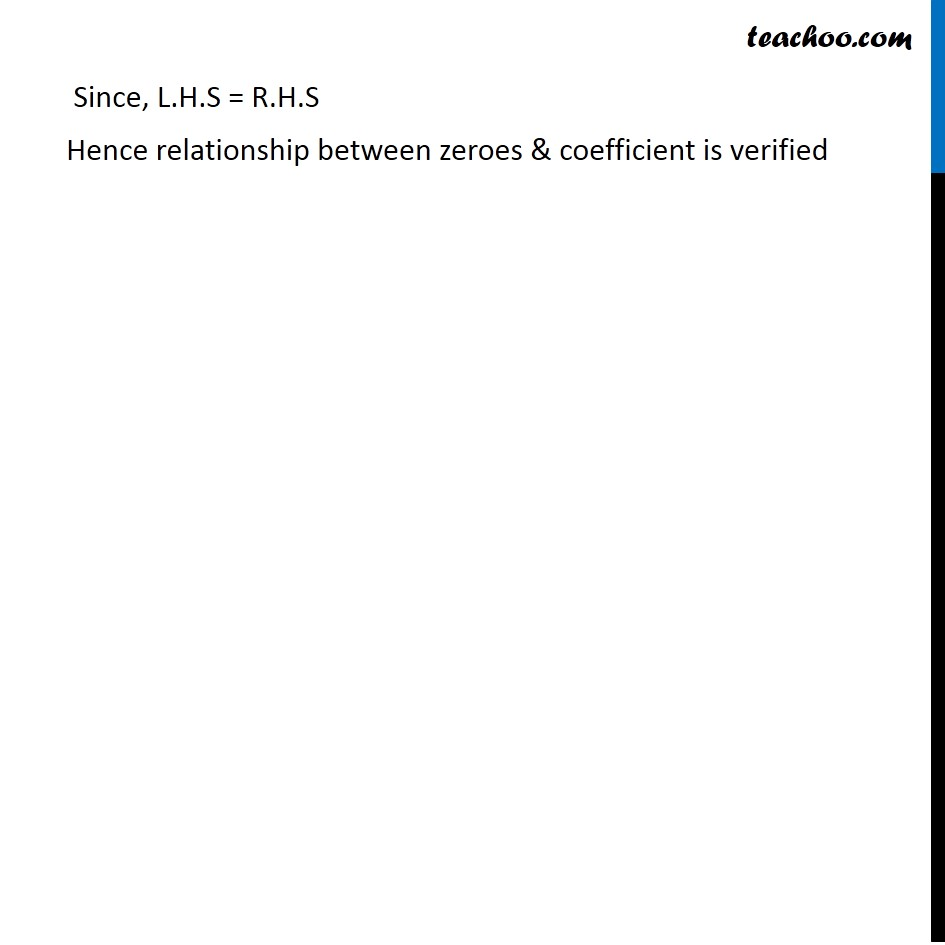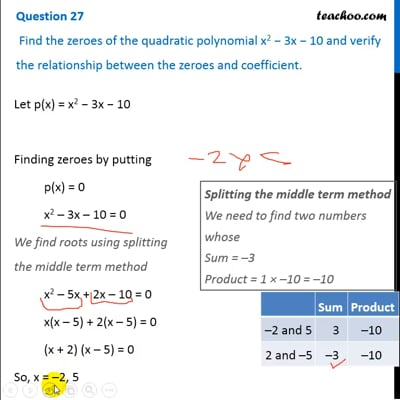CBSE Class 10 Sample Paper for 2020 Boards - Maths Basic

Class 10
Solutions of Sample Papers for Class 10 Boards

## Find the zeroes of the quadratic polynomial x 2 − 3x − 10 and verify the relationship between the zeroes and coefficient.This video is only available for Teachoo black users

Note : This is similar to Ex 2.2, 1 of NCERT – Chapter 2 Class 10

Introducing your new favourite teacher - Teachoo Black, at only ₹83 per month

### Transcript

Question 27 Find the zeroes of the quadratic polynomial x2 − 3x − 10 and verify the relationship between the zeroes and coefficient. Let p(x) = x2 − 3x − 10 Finding zeroes by putting p(x) = 0 x2 – 3x – 10 = 0 We find roots using splitting the middle term method x2 – 5x + 2x – 10 = 0 x(x – 5) + 2(x – 5) = 0 (x + 2) (x – 5) = 0 So, x = –2, 5 Splitting the middle term method We need to find two numbers whose Sum = –3 Product = 1 × –10 = –10 So, zeroes are –2 and 5 Now, lets verify the relationship p(x) = x2 − 3x − 10 Sum of zeroes Sum of zeroes = − (𝐶𝑜𝑒𝑓𝑓𝑖𝑐𝑖𝑒𝑛𝑡 𝑜𝑓 𝑥)/(𝐶𝑜𝑒𝑓𝑓𝑖𝑐𝑖𝑒𝑛𝑡 𝑜𝑓 𝑥2) LHS –2 + 5 = 3 RHS (−𝑏)/𝑎 = (−(−3))/1 = 3 Product of zeroes Product of zeroes = (𝐶𝑜𝑛𝑠𝑡𝑎𝑛𝑡 𝑡𝑒𝑟𝑚)/(𝐶𝑜𝑒𝑓𝑓𝑖𝑐𝑖𝑒𝑛𝑡 𝑜𝑓 𝑥2) LHS –2 × 5 = –10 RHS 𝑐/𝑎 = (−10)/1 = –10 Since, L.H.S = R.H.S Hence relationship between zeroes & coefficient is verified